### Home > A2C > Chapter 13 > Lesson 13.2.3 > Problem13-136

13-136.
1. Without using a calculator, solve these two problems from a college entrance exam.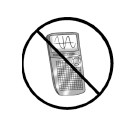Homework Help ✎

1. If x−4/3 + 1 = 17, find x−1/3.

2. If log(A) = a, find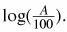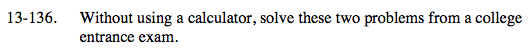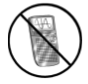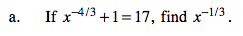Subtract 1 from both sides.

$\small x^{-\frac{4}{3}}=16$

$\text{Raise both sides to the} -\frac{3}{4}\text{ power.}$

$\text{Now raise }\frac{1}{8}\text{ to the }-\frac{1}{3}\text{ power.}$

2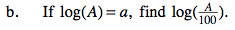Simplify the second log.

log(A) − log(100)

Remember that 100 is 10 to the 2nd power.

log(A) − 2

a − 2